## Introduction

In this lecture we consider the basics of machine learning in neural networks.

## An Artificial Neuron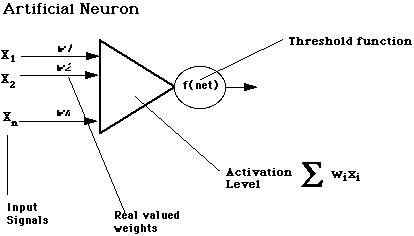## Connectionist Learning

### Hebbian Learning (1949):

Repeated stimulation between two or more neurons strengthens the connection weights among those neurons. One problem with this model is it had no way to model inhibition between neurons.

### Perceptron Learning (1958):

A perceptron is a single-layer network that calculates a linear combination of its inputs and outputs a 1 if the result is greater than some threshold and a -1 if it is not: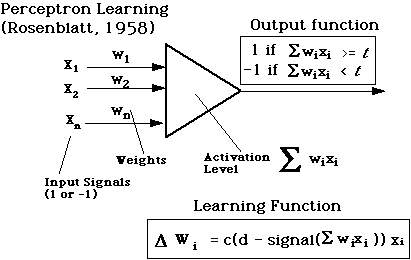### Supervised Perceptron Learning

• c : learning-rate parameter
• d: desired output value
• signal() is the perceptron's actual output value, which is always +1 or -1
• cases
1. d - signal = 0 ==> do nothing
2. d - signal = +2 ==> increment wi by 2cxi
3. d - signal = -2==> decrement wi by 2cxi

By repeatedly adjusting weights in this fashion for an entire set of training data, the perceptron will minimize the average error over the entire set.

Minsky and Papert (1969) showed that if there is a set of weights that give the correct output for an entire training set, a perceptron will learn it.

Example: Perceptrons can learn models for the following primitive boolean functions: AND, OR, NOT, NAND, NOR. Here's an example for AND: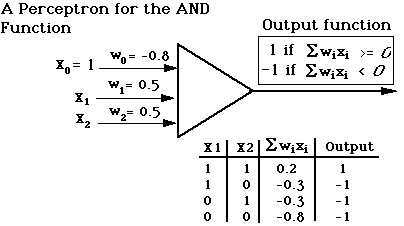### Limitations of Perceptrons

Minsky and Papert (1969) showed that perceptrons could not model the exclusive-or function, because its outputs are not linearly separable. Two classes of outputs are linearly separable if and only if you can draw a straight line in two dimensions that separates one classification from another.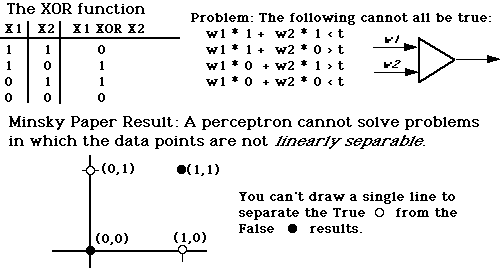## The Delta Rule (Rumelhart, 1986)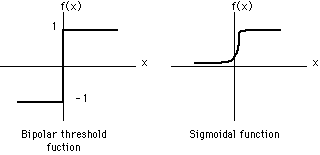The perceptron activation function is a hard-limiting threshold function. A more general neural network uses a continuous activation function. One popular function is the sigmoidal (s-shaped) function, such as the logistic function:

f(net) = 1/(1 + e-L*net)

where L is lambda, a parameter for "squashing" the function and net is the output or sum of the weights.

The delta rule is a learning rule for a network with a continuous (and therefore differentiable) activation function. It attempts to minimize the cumulative error over a data set as a function of the weights in the network:

Delta(wji) = c(di - Oi)f'(neti)xj

where c is the learning rate, di and Oi are the desired and actual outputs for the ith node, and f'(net) is the derivative of the activation function for the ith node, and xj is the jth input to the ith node.

Key Point: The delta rule is tries to minimize the slope of the cumulative error in a particular region of the network's output function. This makes is susceptible to local minima.

## Back propagation Learning for Multilayer Networks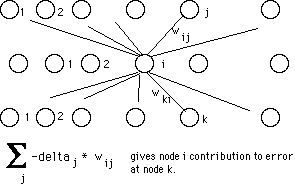Back propagation starts at the output layer and propagates the error backwards through the network. The learning rule is often called the generalized delta rule.

Back propagation activation function is the logistic function:

f(net) = 1/(1 + e-L*net)

The logistic function is useful for assigning error to the hidden layers in a multi-layer network because:

• It is continuous and has a derivative everywhere.
• It is sigmoidal.
• The derivative is greatest where the function is steepest. This assigns the most error to nodes whose activation is least certain.

The formulas for computing the adjustments of the kth weight of the ith node:

Delta(wik) = -c(di - Oi) * Oi(1 - Oi)xik
for nodes on the output layer

Delta(wik) = -c * Oi(1 - Oi)Sum(-deltaj * wij)xik
for nodes on the hidden layers.

## NETtalk System (Sejnowski and Rosenberg, 1987)

Nettalk is a neural network, developed in 1987, that learns to pronounce English text. It learns to associate phonemes with string of text.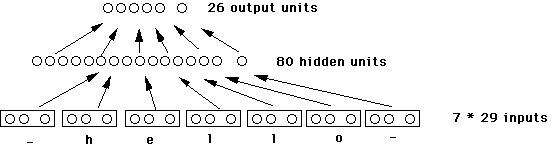### Properties of NETtalk

• Learned to pronounce English text.
• Inputs: String of text, e.g. "I say hello to you" (7 letter window)
• Input Unit: 29 units, one for each letter and 3 for punctuation and spaces
• Outputs: Phonemes (26 different ones)
• Hidden Elements: 80 (These units learn the pronounciation rules)
• Connections: 18,629
• Learning rule: back propagation
• Interesting Properties
• Performance improves with training but at a slower rate.
• Relearning was highly efficient

### NETtalk Comparison with ID3 (Shavlik, 1991)

• Both ID3 and NETtalk were able to pronounce 60% after 500 training examples
• ID3 required 1 pass through the training data
• NETtalk was allowed 100 passes through the 500 training data

## Using Encog Java Neural Network Framework

• encog-workbench-3.0.1-release.zip
• encog-examples-3.0.1-release.zip
• encog-core-3.0.1-release.zip

Exercises

1. Take a look at the Getting Started Documentation.

2. Command Line Exercise: Do the Encog Java XORHelloWorld example. Try working through the ANT version. On my system, this is the Java command you need to run from within the .../encog-examples-3.0.1/lib:
```java -cp encog-core-3.0.1-SNAPSHOT.jar:examples.jar org.encog.examples.neural.xor.XORHelloWorld
```

3. GUI Exercise: Do the Workbench Classification Example.